Next: Specific heats of solids Up: Applications of statistical thermodynamics Previous: Specific heats

## Specific heats of gases

Let us now investigate the specific heats of gases. Consider, first of all, translational degrees of freedom. Every molecule in a gas is free to move in three dimensions. If one particular molecule has massand momentum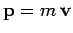then its kinetic energy of translation is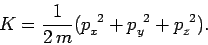(492)

The kinetic energy of other molecules does not involve the momentum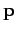of this particular molecule. Moreover, the potential energy of interaction between molecules depends only on their position coordinates, and, thus, certainly does not involve. Any internal rotational, vibrational, electronic, or nuclear degrees of freedom of the molecule also do not involve. Hence, the essential conditions of the equipartition theorem are satisfied (at least, in the classical approximation). Since Eq. (492) contains three independent quadratic terms, there are clearly three degrees of freedom associated with translation (one for each dimension of space), so the translational contribution to the molar heat capacity of gases is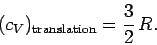(493)

Suppose that our gas is contained in a cubic enclosure of dimensions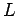. According to Schrödinger's equation, the quantized translational energy levels of an individual molecule are given by(494)

where,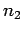, andare positive integer quantum numbers. Clearly, the spacing between the energy levels can be made arbitrarily small by increasing the size of the enclosure. This implies that translational degrees of freedom can be treated classically, so that Eq. (493) is always valid (except very close to absolute zero). We conclude that all gases possess a minimum molar heat capacity ofdue to the translational degrees of freedom of their constituent molecules.

The electronic degrees of freedom of gas molecules (i.e., the possible configurations of electrons orbiting the atomic nuclei) typically give rise to absorption and emission in the ultraviolet or visible regions of the spectrum. It follows from Tab. 3 that electronic degrees of freedom are frozen out at room temperature. Similarly, nuclear degrees of freedom (i.e., the possible configurations of protons and neutrons in the atomic nuclei) are frozen out because they are associated with absorption and emission in the X-ray and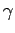-ray regions of the electromagnetic spectrum. In fact, the only additional degrees of freedom we need worry about for gases are rotational and vibrational degrees of freedom. These typically give rise to absorption lines in the infrared region of the spectrum.

The rotational kinetic energy of a molecule tumbling in space can be written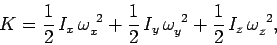(495)

where the-,-, and-axes are the so called principle axes of inertia of the molecule (these are mutually perpendicular),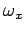,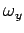, andare the angular velocities of rotation about these axes, and,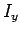, andare the moments of inertia of the molecule about these axes. No other degrees of freedom depend on the angular velocities of rotation. Since the kinetic energy of rotation is the sum of three quadratic terms, the rotational contribution to the molar heat capacity of gases is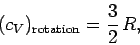(496)

according to the equipartition theorem. Note that the typical magnitude of a molecular moment of inertia is, whereis the molecular mass, and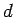is the typical interatomic spacing in the molecule. A special case arises if the molecule is linear (e.g. if the molecule is diatomic). In this case, one of the principle axes lies along the line of centers of the atoms. The moment of inertia about this axis is of order, whereis a typical nuclear dimension (remember that nearly all of the mass of an atom resides in the nucleus). Since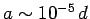, it follows that the moment of inertia about the line of centres is minuscule compared to the moments of inertia about the other two principle axes. In quantum mechanics, angular momentum is quantized in units of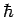. The energy levels of a rigid rotator are written(497)

whereis the moment of inertia andis an integer. Note the inverse dependence of the spacing between energy levels on the moment of inertia. It is clear that for the case of a linear molecule, the rotational degree of freedom associated with spinning along the line of centres of the atoms is frozen out at room temperature, given the very small moment of inertia along this axis, and, hence, the very widely spaced rotational energy levels.

Classically, the vibrational degrees of freedom of a molecule are studied by standard normal mode analysis of the molecular structure. Each normal mode behaves like an independent harmonic oscillator, and, therefore, contributesto the molar specific heat of the gas [from the kinetic energy of vibration andfrom the potential energy of vibration]. A molecule containing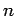atoms has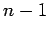normal modes of vibration. For instance, a diatomic molecule has just one normal mode (corresponding to periodic stretching of the bond between the two atoms). Thus, the classical contribution to the specific heat from vibrational degrees of freedom is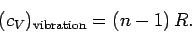(498)So, do any of the rotational and vibrational degrees of freedom actually make a contribution to the specific heats of gases at room temperature, once quantum effects are taken into consideration? We can answer this question by examining just one piece of data. Figure 3 shows the infrared absorption spectrum of Hydrogen Chloride. The absorption lines correspond to simultaneous transitions between different vibrational and rotational energy levels. Hence, this is usually called a vibration-rotation spectrum. The missing line at about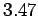microns corresponds to a pure vibrational transition from the ground-state to the first excited state (pure vibrational transitions are forbidden: HCl molecules always have to simultaneously change their rotational energy level if they are to couple effectively to electromagnetic radiation). The longer wavelength absorption lines correspond to vibrational transitions in which there is a simultaneous decrease in the rotational energy level. Likewise, the shorter wavelength absorption lines correspond to vibrational transitions in which there is a simultaneous increase in the rotational energy level. It is clear that the rotational energy levels are more closely spaced than the vibrational energy levels. The pure vibrational transition gives rise to absorption at aboutmicrons, which corresponds to infrared radiation of frequency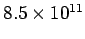hertz with an associated radiation temperature'' of 4400 degrees kelvin. We conclude that the vibrational degrees of freedom of HCl, or any other small molecule, are frozen out at room temperature. The rotational transitions split the vibrational lines by aboutmicrons. This implies that pure rotational transitions would be associated with infrared radiation of frequency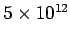hertz and corresponding radiation temperature'' 260 degrees kelvin. We conclude that the rotational degrees of freedom of HCl, or any other small molecule, are not frozen out at room temperature, and probably contribute the classicalto the molar specific heat. There is one proviso, however. Linear molecules (like HCl) effectively only have two rotational degrees of freedom (instead of the usual three), because of the very small moment of inertia of such molecules along the line of centres of the atoms.

We are now in a position to make some predictions regarding the specific heats of various gases. Monatomic molecules only possess three translational degrees of freedom, so monatomic gases should have a molar heat capacityjoules/degree/mole. The ratio of specific heats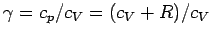should be. It can be seen from Tab. 2 that both of these predictions are borne out pretty well for Helium and Argon. Diatomic molecules possess three translational degrees of freedom and two rotational degrees of freedom (all other degrees of freedom are frozen out at room temperature). Thus, diatomic gases should have a molar heat capacityjoules/degree/mole. The ratio of specific heats should be. It can be seen from Tab. 2 that these are pretty accurate predictions for Nitrogen and Oxygen. The freezing out of vibrational degrees of freedom becomes gradually less effective as molecules become heavier and more complex. This is partly because such molecules are generally less stable, so the force constant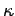is reduced, and partly because the molecular mass is increased. Both these effect reduce the frequency of vibration of the molecular normal modes [see Eq. (472)], and, hence, the spacing between vibrational energy levels [see Eq. (471)]. This accounts for the obviously non-classical [i.e., not a multiple of] specific heats of Carbon Dioxide and Ethane in Tab. 2. In both molecules, vibrational degrees of freedom contribute to the molar specific heat (but not the fullbecause the temperature is not high enough).Figure 4 shows the variation of the molar heat capacity at constant volume (in units of) of gaseous hydrogen with temperature. The expected contribution from the translational degrees of freedom is(there are three translational degrees of freedom per molecule). The expected contribution at high temperatures from the rotational degrees of freedom is(there are effectively two rotational degrees of freedom per molecule). Finally, the expected contribution at high temperatures from the vibrational degrees of freedom is(there is one vibrational degree of freedom per molecule). It can be seen that as the temperature rises the rotational, and then the vibrational, degrees of freedom eventually make their full classical contributions to the heat capacity.Next: Specific heats of solids Up: Applications of statistical thermodynamics Previous: Specific heats
Richard Fitzpatrick 2006-02-02